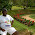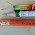## Tuesday, December 8, 2009

### Problem 400. Triangle, Angle bisector, Circumcircle, Perpendicular, Congruence

Proposed Problem
Click the figure below to see the complete problem 400 about Triangle, Angle bisector, Circumcircle, Perpendicular, Congruence.Complete Problem 400
Level: High School, SAT Prep, College geometry

1.|OG|=|OH|
|OC|=|OD|=r
|GC|=|DH|

2.Reasons for step 1: |OG|=|OH| ?

3.suggest to others

1) draw HP perpendicular to AC ( P on AC )
2) draw OK perpendicular to HG ( K on HG )

about first comment: step 1 and step 2 are not enough for step 3

4.reason for step 1:
http://i49.tinypic.com/69omdj.gif

and
step 1 and step 2 are enough for step 3, i think

5.6.W/o having to refer to any other figure or any construction, we can easily see that quad. OFCE is concyclic and hence ang. HOG = ang. C while ang. OGH = ang. EGC = 90-C/2. Therefore, ang. OHG = 180 – C - (90-C/2)= 90 – C/2 or ang. OHG = ang. OGH. Hence etc.
Ajit

7.step 1 and 2 need third condition of congruence,
angle DOH = angle COG ? verify please

my solution
to draw OK perpendicular to HG give
1) OK is median => HK = KG
2) OK is diameter perpendicular to chord DC => DK=KC
so DH=DK-HK
and GC=KC-KG

8.Triangles HFC and EGC are similar

So OH = OG

So Tr.s ODH and OGC are congruent ASA and the result follows

Sumith Peiris
Moratuwa
Sri Lanka

9.OGH isosceles ==>
perpendicular from O to CD bisect GH, and also bisect CD ==>
GC = DH

10.More or less the same as the last few but with a few extra steps included
1. Right triangle CEG is similar to right triangle CFH because of the angle bisector.
2. So angle CGE = angle HGO = angle FHC and triangle OHG is isosceles.
3. OD = OC since they are radii.
4. So CDO is isoscleses and angle ODC = OCD.
5. Angle OHD = OGC since they are supplementary to 2 congruent angles.
6. We now have 2 out of 3 angles and 2 out of 3 sides congruent in ODH and OGC which is more than enough to infer a missing angle and use either SAS or ASA to show the triangles are congruent.
7. So DH = CG.